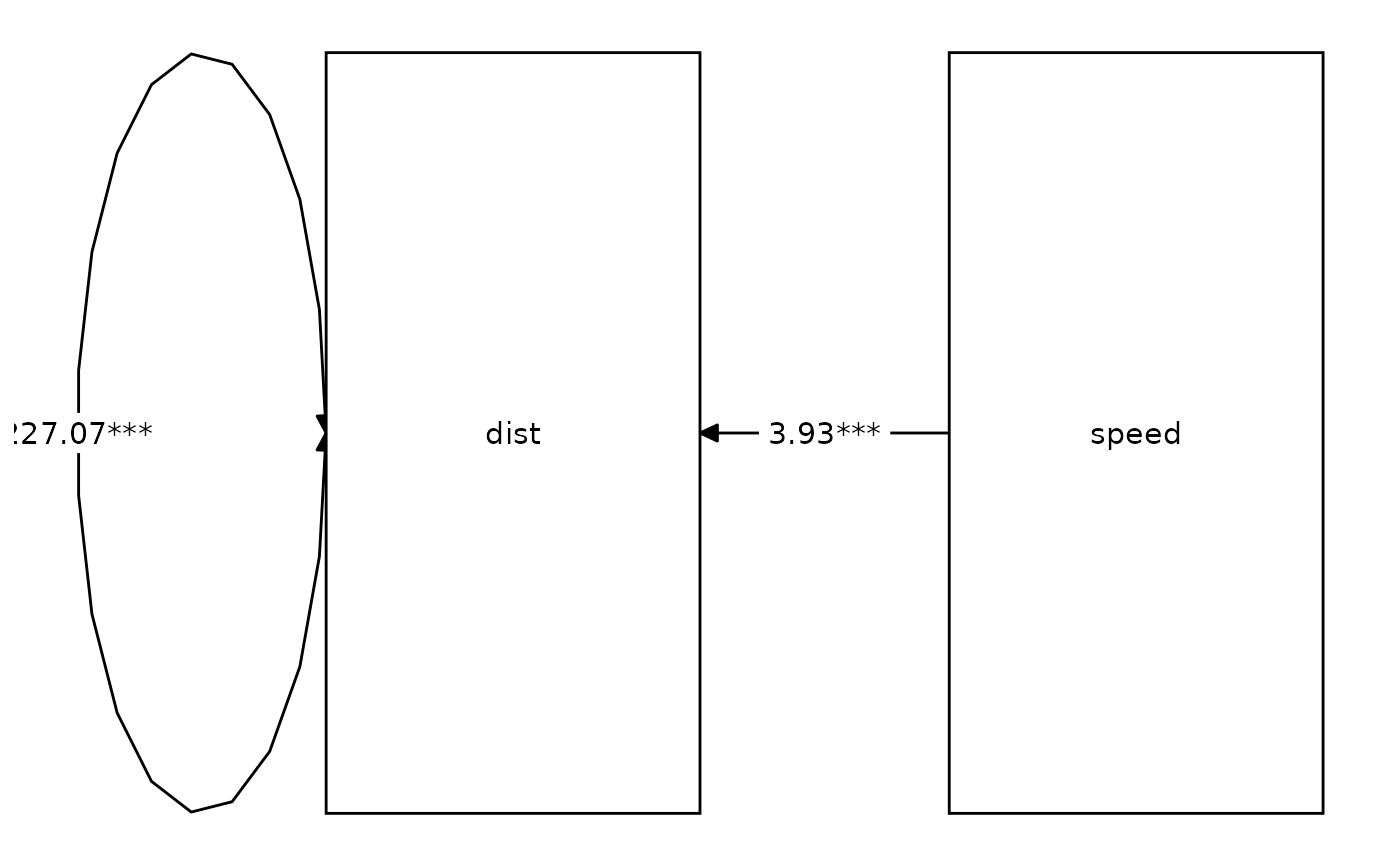Render a graph based on a layout, and either nodes and edges, or a model object.

# S3 method for dagitty
graph_sem(model, ...)

graph_sem(...)

# S3 method for default
graph_sem(
edges = NULL,
layout = NULL,
nodes = NULL,
rect_width = 1.2,
rect_height = 0.8,
ellipses_width = 1,
ellipses_height = 1,
variance_diameter = 0.8,
spacing_x = 2,
spacing_y = 2,
text_size = 4,
curvature = 60,
angle = NULL,
fix_coord = FALSE,
...
)

# S3 method for lavaan
graph_sem(model, edges = NULL, layout = NULL, nodes = NULL, ...)

# S3 method for MxModel
graph_sem(model, edges = NULL, layout = NULL, nodes = NULL, ...)

# S3 method for mplus.model
graph_sem(model, edges = NULL, layout = NULL, nodes = NULL, ...)

# S3 method for character
graph_sem(...)

# S3 method for mplusObject
graph_sem(model, edges = NULL, layout = NULL, nodes = NULL, ...)

## Arguments

model

Instead of the edges argument, it is also possible to use the model argument and pass an object for which a method exists (e.g., mplus.model or lavaan).

...

Additional arguments passed to and from functions.

edges

Object of class 'tidy_edges', or a data.frame with (at least) the columns c("from", "to"), and optionally, c("arrow", "label", "connect_from", "connect_to", "curvature").

layout

A matrix (or data.frame) that describes the layout; see get_layout.

nodes

Optional, object of class 'tidy_nodes', created with the get_nodes function, or a data.frame with (at least) the column c("name"), and optionally, c("shape", "label"). If set to NULL (the default), nodes are inferred from the layout and edges arguments.

rect_width

Width of rectangles (used to display observed variables), Default: 1.2

rect_height

Height of rectangles (used to display observed variables), Default: 0.8

ellipses_width

Width of ellipses (used to display latent variables), Default: 1

ellipses_height

Height of ellipses (used to display latent variables), Default: 1

variance_diameter

Diameter of variance circles, Default: .8

spacing_x

Spacing between columns of the graph, Default: 1

spacing_y

Spacing between rows of the graph, Default: 1

text_size

Point size of text, Default: 4

curvature

Curvature of curved edges. The curve is a circle segment originating in a point that forms a triangle with the two connected points, with angles at the two connected points equal to curvature. To flip a curved edge, use a negative value for curvature. Default: 60

angle

Angle used to connect nodes by the top and bottom. Defaults to NULL, which means Euclidean distance is used to determine the shortest distance between node sides. A numeric value between 0-180 can be provided, where 0 means that only nodes with the same x-coordinates are connected top-to-bottom, and 180 means that all nodes are connected top-to-bottom.

fix_coord

Whether or not to fix the aspect ratio of the graph. Does not work with multi-group or multilevel models. Default: FALSE.

## Value

Object of class 'sem_graph'

## Details

The default interface simply Runs the functions prepare_graph and plot. The alternative interface first runs get_nodes and get_edges on the model argument.

## Examples

library(lavaan)
res <- sem("dist ~ speed", cars)
graph_sem(res)